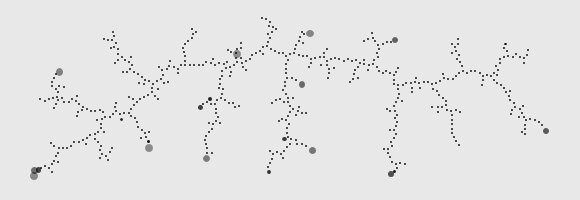### Neural Particle DepositionIn developing an algorithm to generate nodes that are guaranteed to be within a certain distance of each other, I stumbled over this interesting distribution of particles which were initially placed randomly then moved near their nearest neighbor. I’ve dubbed this particle system “Neural Particle Deposition” on account of the organic, neuron-like shapes it produces. Take a look:

This algorithm is rather straightforward: after picking the starting point, generate one point at a time within the container’s constraints. If the generated point is too close to its closest point, drop it. Otherwise, move it along the vector to its closest neighbor so it is a certain maximum distance away, then repeat.

The code relies on a container div with the ID of “canvas”, which must have an explicit width and height defined, as well as its position attribute set to “relative”, so that the pips can be absolutely positioned within it. The placed pips are will be given the class of “pip”, with the following initial style:

```.pip
{
width: 8px;
height: 8px;
background-color: #000000;
position: absolute;
opacity: 0.4;
}
```

The JavaScript code is as follows:

```\$(function()
{
var newPipInterval = 8;

// The farthest
var pipCount = 600;

var center;

var canvas = \$("#canvas");
var width = canvas.width();
var height = canvas.height();

var pips = new Array();

reset();

function reset ()
{
canvas.empty();
pips = new Array();
center = createRandomPip();
}

{
if (pips.length < pipCount)
{
}
else
{
reset();
}
}

function createPip ()
{
var randomPip = createRandomPip();
var nearestPip = getNearestPip(randomPip);
var pipDistance = distance(randomPip, nearestPip);

// Too close, toss
{
randomPip = createRandomPip();
nearestPip = getNearestPip(randomPip);
pipDistance = distance(randomPip, nearestPip);
}

// Adjust if we're too far
{
// Calculate unit vector
var unitX = (randomPip.x - nearestPip.x) / pipDistance;
var unitY = (randomPip.y - nearestPip.y) / pipDistance;

randomPip.x = avgPipRadius * unitX + nearestPip.x;
randomPip.y = avgPipRadius * unitY + nearestPip.y;
}

return randomPip;
}

function getNearestPip (pip)
{
var nearestPip = center;
var nearestDistance = distance(pip, center);

for (var i = 0; i < pips.length; i++)
{
var candidatePip = pips[i];
var candidateDistance = distance(pip, candidatePip);

if (candidateDistance < nearestDistance)
{
nearestPip = candidatePip;
nearestDistance = candidateDistance;
}
}

return nearestPip;
}

function createRandomPip ()
{
return {x: rand(0, width), y: rand(0, height)};
}

{
pips.push(pip);

var div = document.createElement("div");
div.setAttribute("class", "pip");
div.style.left = pip.x + "px";
div.style.top = pip.y + "px";

canvas.append(div);

\$(div).animate({width: "2px", height: "2px", opacity: 1}, 500);
}

function rand(min, max)
{
return Math.floor(Math.random()*(max-min+1)+min);
}

function clamp (number, min, max)
{
return Math.min(Math.max(number, min), max);
}

function distance(pip1, pip2)
{
var xs = 0;
var ys = 0;

xs = pip2.x - pip1.x;
xs = xs * xs;

ys = pip2.y - pip1.y;
ys = ys * ys;

return Math.sqrt(xs + ys);
}
});
```

You can tame the distribution somewhat by altering the container’s dimensions, as you can see in the header image. To generate a more reliable mountain-range-like distribution, try starting with a narrow container and setting the starting position to one of the ends.

The full source code is available on GitHub as well as Google Code. Enjoy!